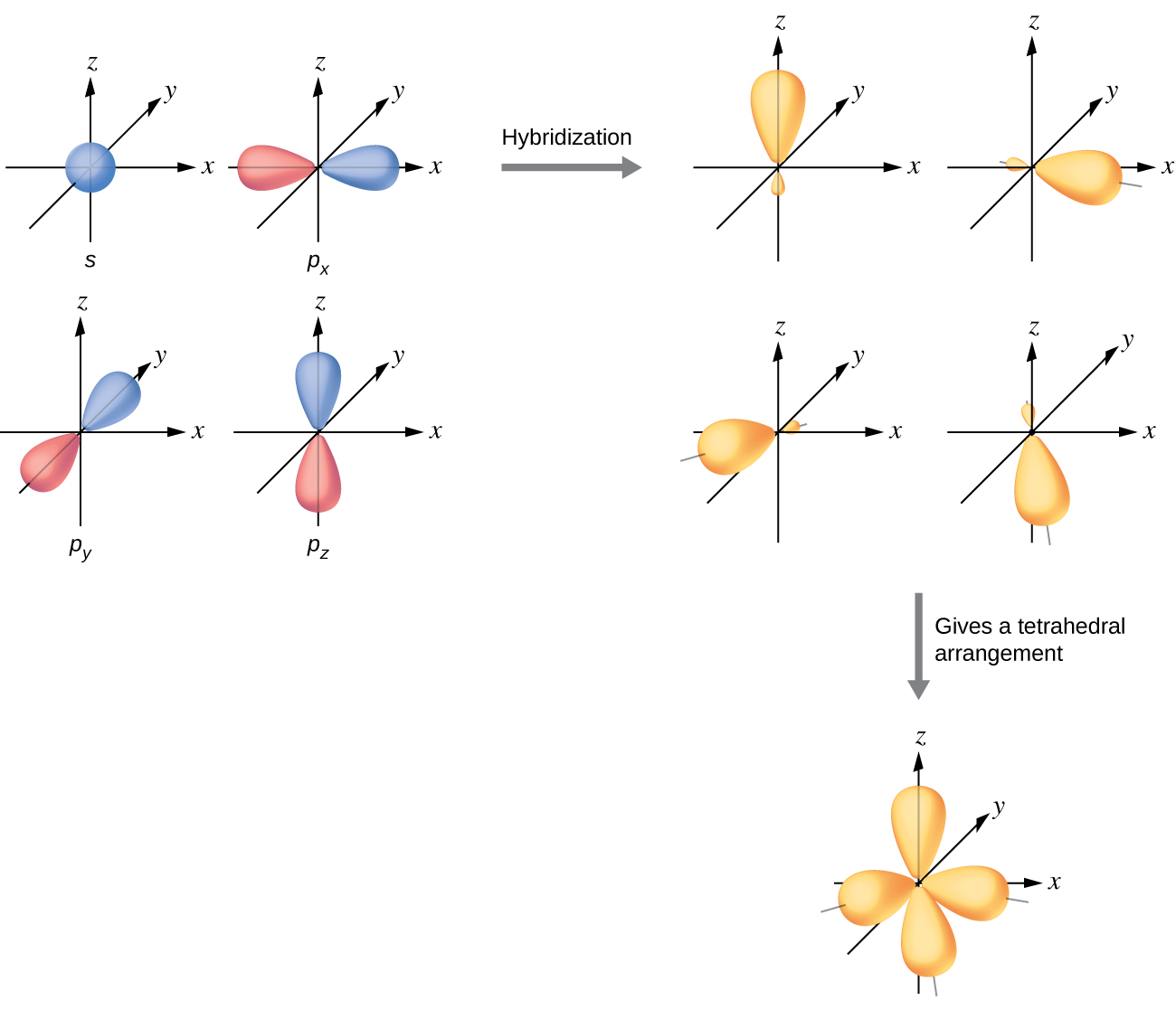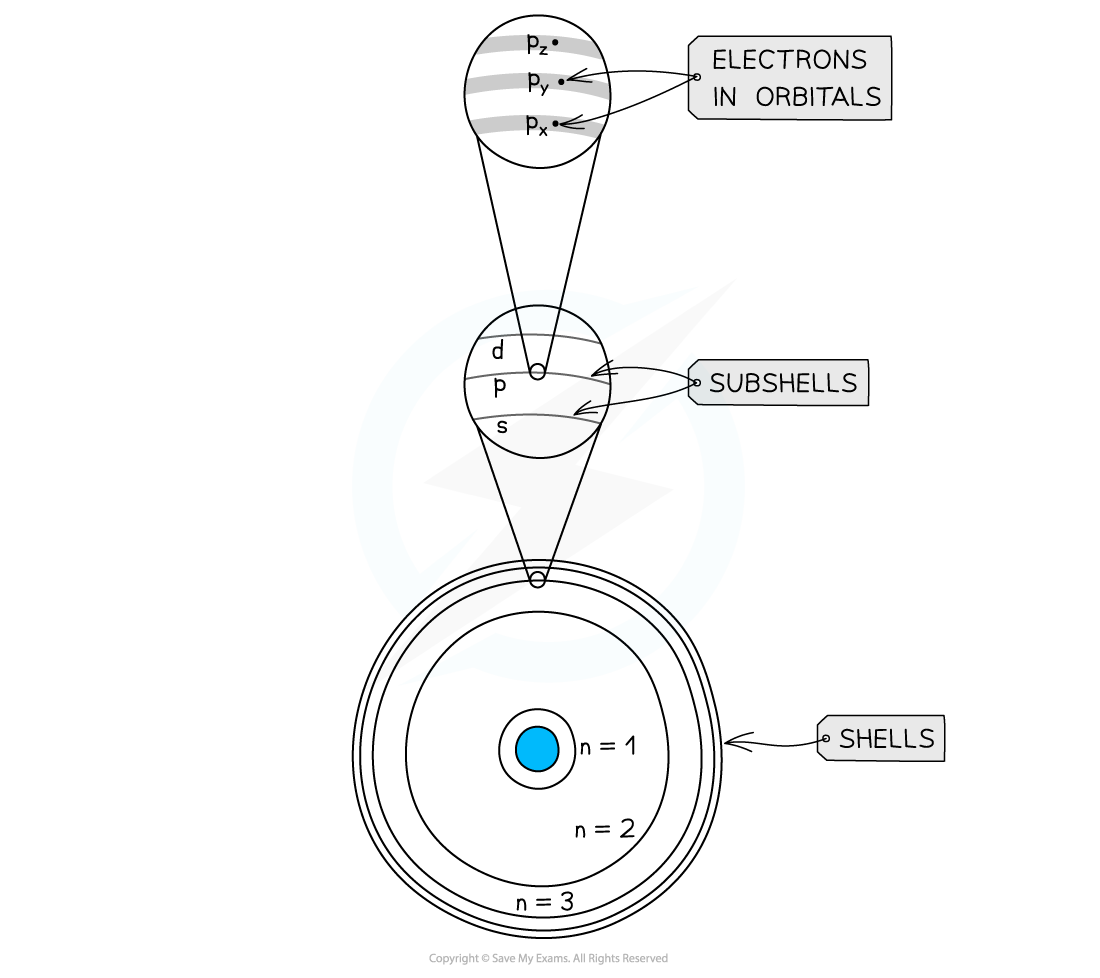Have you ever thought about how tiny atoms are? They're so small that it would take one million of them to be as thick as a sheet of paper. That's a lot of atoms! Atoms have a nucleus, which is full of protons and neutrons. Most of the atom is empty space, but there are also electrons that orbit around the nucleus. These orbits are called electron shells or energy levels. The shells are numbered based on their distance from the nucleus, with the first shell being the closest. The higher the number, the further away the shell is from the nucleus and the more energy it has. Each shell can hold a certain number of electrons, based on its principal quantum number. For example, the second shell can hold eight electrons. Understanding electron shells is important for understanding atomic structure and reactivity.

## What are electron sub-shells?

Electron shells are split into smaller sub-shells which themselves contain orbitals. We’ll explore subshells together first before moving on to orbitals.

### Sub-shell types

Each electron shell, also known as an energy level, has sub-levels, also known as sub-shells. Sub-shells are like mini divisions within each shell. The four types of sub-shells are s, p, d, and f. However, not every shell has each type of sub-shell. For example, the shell closest to the nucleus, with n = 1, only has an s sub-shell called 1s. The second shell has sub-shells 2s and 2p, while the third shell has sub-shells 3s, 3p, and 3d.

### Sub-shell energy levels

Each electron shell has its own energy level, and as the principal quantum number increases, the energy level of the shell increases too. Similarly, each sub-shell within a shell has a different energy level, with s sub-shells having the lowest energy level, followed by p, d, and f. It's important to remember that all the sub-shells in one electron shell have a lower energy level than the sub-shells in a higher energy shell with a higher principal quantum number. However, there is one exception to this rule. Sub-shell 3d has a higher energy level than 4s, even though it's in a shell with a lower principal quantum number.

## What are electron orbitals?

Orbitals are regions of space where electrons can be found 95 percent of the time. Electrons can act like both particles and waves, depending on whether they are being observed or not. This is a concept in quantum mechanics, and in 1925, Erwin Schrödinger developed an equation to predict the location and energy of an electron based on its behavior as a wave. This equation earned him a Nobel prize for physics in 1933. Taking the example of hydrogen, which has one electron, if you repeatedly plot the electron's location, you would eventually end up with a sketch that resembles an orbital.

We know this region as the orbital from the sub-shell 1s. As you can see, this orbital is roughly spherical. Let’s take a closer look at the shapes and properties of all the other orbitals.

### Orbital shapes

Orbitals have different shapes, depending on their sub-shell. S orbitals are spherical, p orbitals are a figure of eight, and d orbitals can have a variety of shapes.A diagram showing the shapes of the s orbital, left, and the p orbital, right

All orbitals can hold a maximum of 2 electrons, but they can have fewer than 2. The number of electrons an orbital can hold is influenced by the number of orbitals within a sub-shell. S sub-shells have only one orbital, while p sub-shells have three and d sub-shells have five. This means that s sub-shells can hold a maximum of two electrons, p sub-shells can hold up to six electrons, and d sub-shells can hold up to ten electrons. It's worth noting that f sub-shells have seven orbitals, so they can hold up to 14 electrons. However, this information is beyond the scope of A-level studies.

### Electron spin

The electrons in an orbital must have opposite spins. Spin is a property of electrons that can take it either up or down. In an orbital there can be at most one electron with an up spin, and one with a down spin. (Explore spin more in Understanding NMR.)

### Orbital energy

Orbitals in the same sub-shell all have the same energy. That means, for example, that all 10 electrons in the 3d sub-shell have the same energy as each other; both of the electrons in 2s have the same energy as each other. The following diagram puts together what we know about shells, sub-shells, orbitals, and energy levels to show the quantities and energies of the orbitals up to 4p.A diagram showing the energies of the different electron shells, sub-shells, and orbitals

## Electron configuration

Electrons in an atom are arranged in shells, also known as energy levels. Each shell is assigned a principal quantum number and shells with higher principal quantum numbers are further from the nucleus and have higher energy levels. Electron shells are further divided into sub-levels called sub-shells, which vary in energy level as well. Sub-shells have different numbers of orbitals, which are regions of space where electrons can be found 95 percent of the time. Orbitals can only hold a maximum of two electrons and have different shapes. The arrangement of electrons in shells, sub-shells, and orbitals is known as an element's electron configuration. An atom's electron configuration determines its properties and reactivity. For a more in-depth understanding of electron configuration, refer to the relevant section.

## Electron Shells

What are electron shells?

Electron shells are orbital paths that are followed by electrons around the nucleus of an atom. They’re also known as energy levels.

How many electrons are in each shell?

The first electron shell can hold two electrons. The second can hold eight, and the third 18.

What are the four electron shells?

Electron shells are made up of different types of subshells. The four types of subshells are s, p, d, and f.

What element has six electron shells?

Caesium has six electron shells. In fact, all elements in period 6 in the periodic table have six electron shells.

What are the names of the electron shells?

Electron shells are split into smaller subshells known as s, p, d, and f.14-day free trial. Cancel anytime.Join 20,000+ learners worldwide.The first 14 days are on us96% of learners report x2 faster learningFree hands-on onboarding & supportCancel Anytime